Recently, I am learning how Elliptic Curve Cryptography works. I searched around the internet, found so many articles and videos explaining it. Most of them are covering only a portion of it, some of them skip many critical steps how you get from here to there. In the end, I didn’t find an article that really explains it from end-to-end in an intuitive way. With that in mind, I would like to write a post explaining Elliptic Curve Cryptography, cover from the basics to key exchange, encryption, and decryption.

To plot the curve for writing this article, and also get a sense of how things work, I wrote a Jupyter Notebook for curve plotting and calculations in Python. The plotting library is matplotlib. And if you want to play around an elliptic curve and feel how it works yourself, lucky you! I made the source code open-sourced here on GitHub, one for real numbers and one for finite field: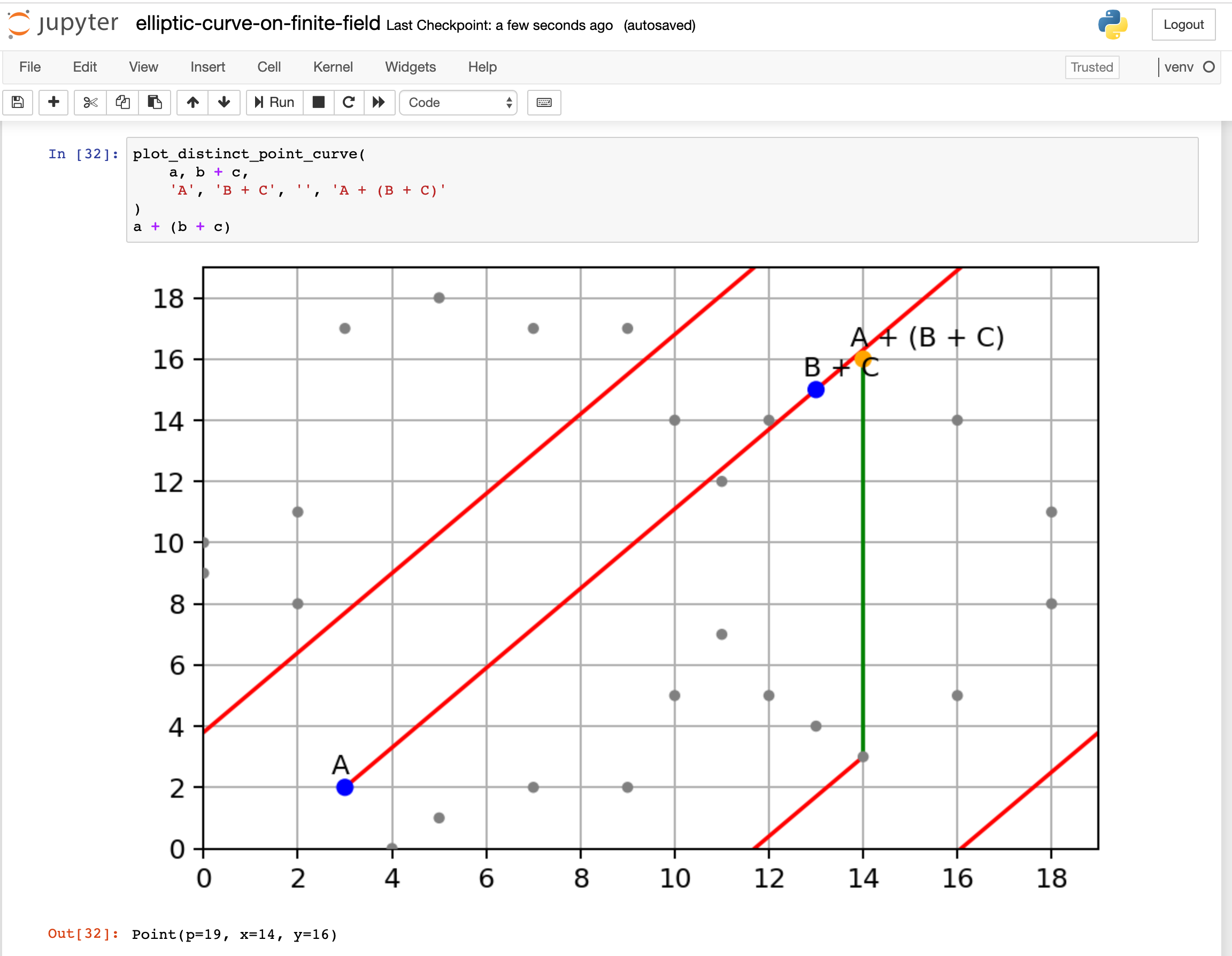Screenshot of my Jupyter notebook for plotting Elliptic Curve

You can find most of the article diagrams in the notebook.

Please note that this article is not meant for explaining how to implement Elliptic Curve Cryptography securely, the example we use here is just for making teaching you and myself easier. We also don’t want to dig too deep into the mathematical rabbit hole, I only want to focus on getting the sense of how it works essentially. So we will strip out many math details and only provide a reference read for it.

Now, shall we begin?Clip from Star Trek Into Darkness movie

## Let’s play a game

An elliptic curve is a curve defined by

$y^{2} = x^{3} + a x + b$

For example, let $$a = -3$$ and $$b = 5$$, then when you plot the curve, it looks like this: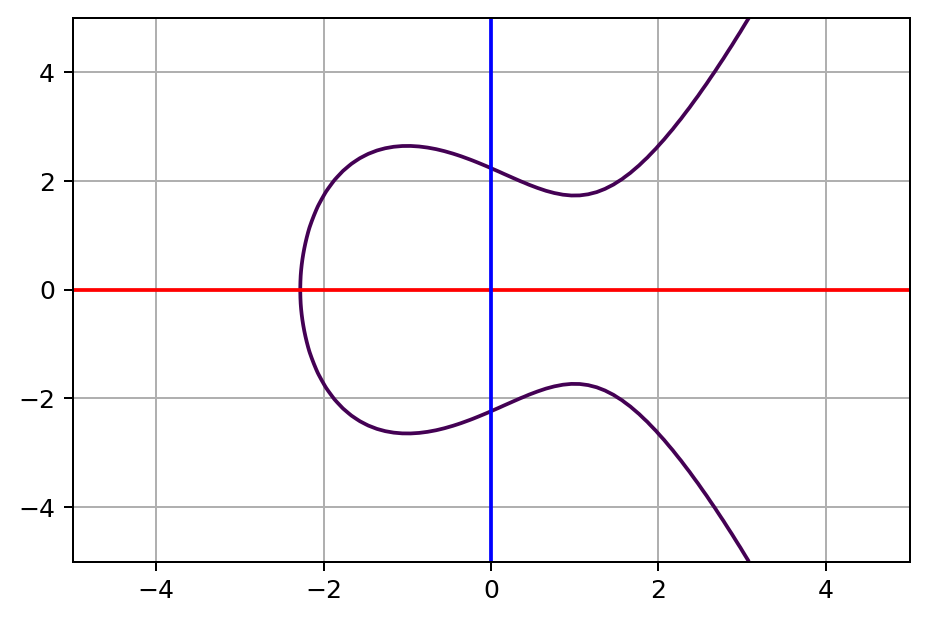A simple elliptic curve

Now, let’s play a game. Pick two different random points with different x value on the curve, connect these two points with a straight line, let’s say $$A$$ and $$B$$. Then you will notice the line touches the curve at a third point. Once we find that third point and flip its y value to the other side of x axis, let’s call it $$A + B$$.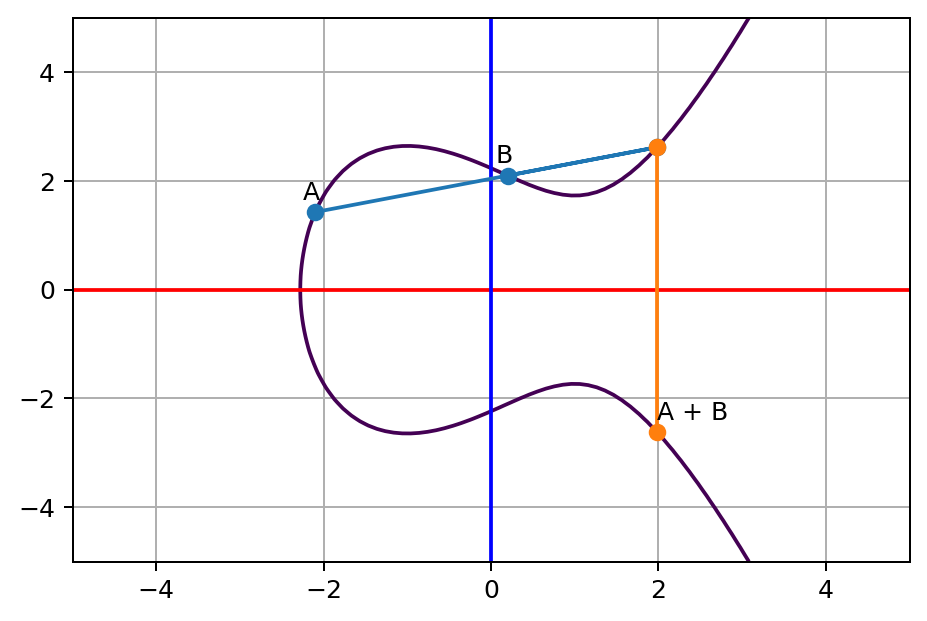A and B line touches a third point on the curve, and its opposite point on the other side of x axis

The point $$A + B$$ is the sum of $$A$$ and $$B$$. You can think of this process is some kind of space travel. Imagine there are enemies following your spacecraft. To lose your enemy, you are taking a straight shortcut off the route to another point on the route, bounce to the other side of the route sharply once you hit the third point.A clip from Star War movie

Well, the enemies still follow, let’s do the trick again. This time we start from last point $$A + B$$ to another point $$C$$.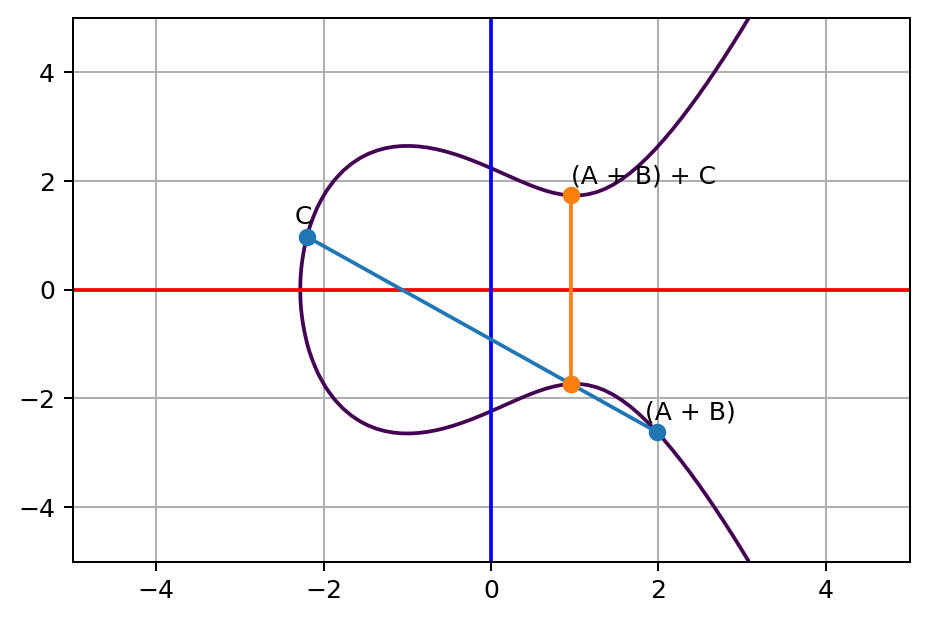A + B and C line touches a third point on the curve, and its opposite point on the other side of x axis

As you can see, we can keep repeating the same trick by adding a new point to bounce to a new sum, as long as the new line is not vertical.

Over time, you realize that it’s tedious to find a new point to jump to. To make the trick more intuitive and easier to repeat, let’s now take the shortcut along the tangent line of our current position $$P$$, and it will look like this: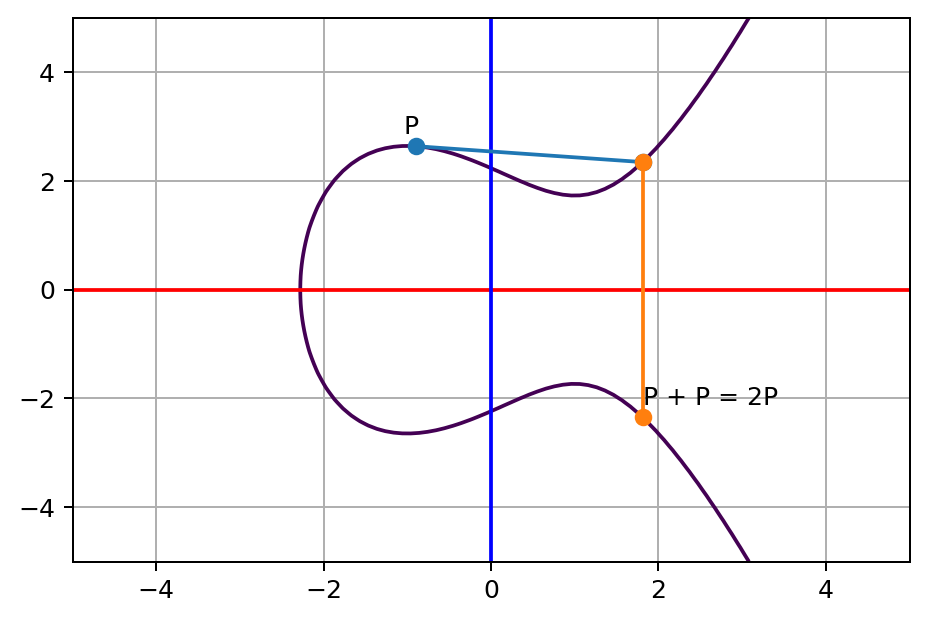tangent line of P touches a third point on the curve, and its opposite point on the other side of x axis

Consider the previous two points style jumping trick, as if you see point $$A$$ and point $$B$$ are infinitely close to each other at $$P$$, it’s actually the same trick. So we can apply the previous rule, call the result sum of $$P$$ and $$P$$, which is $$P + P$$, i.e. $$2P$$.

Likewise, we can repeat the same step more to lose our enemies, this time, we start from $$2P$$ back to the original point $$P$$: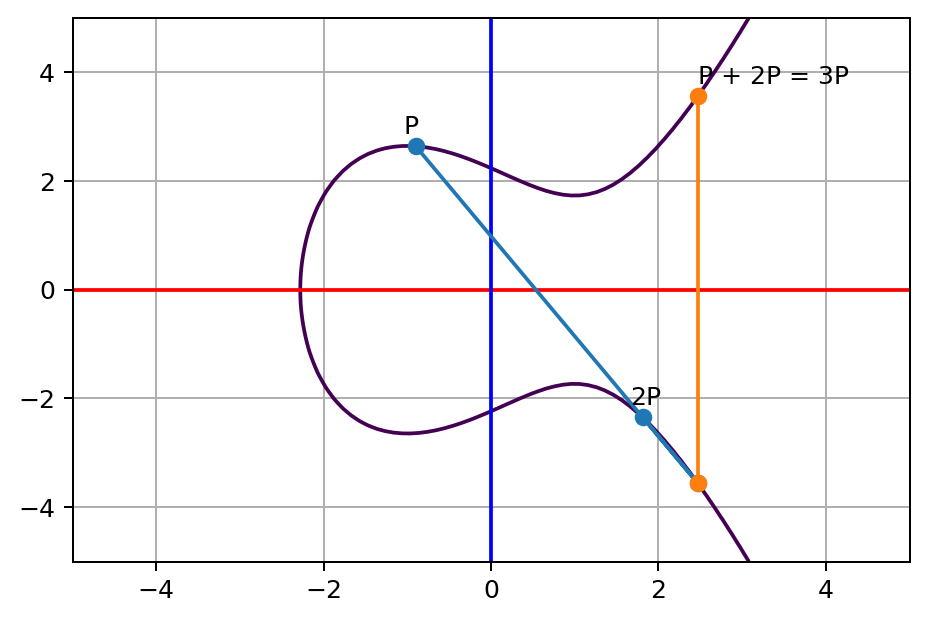tangent line of 2P touches a third point on the curve, and its opposite point on the other side of x axis

For the result, we call it $$P + 2P$$ or $$3P$$. Obviously, we can keep doing this many times to reach $$NP$$. Now, here comes the question, given the coordinate of a point $$NP$$, can you find out what is the $$N$$ value? In other words, how many times we’ve jumped from $$P$$ to $$NP$$, like the following diagram: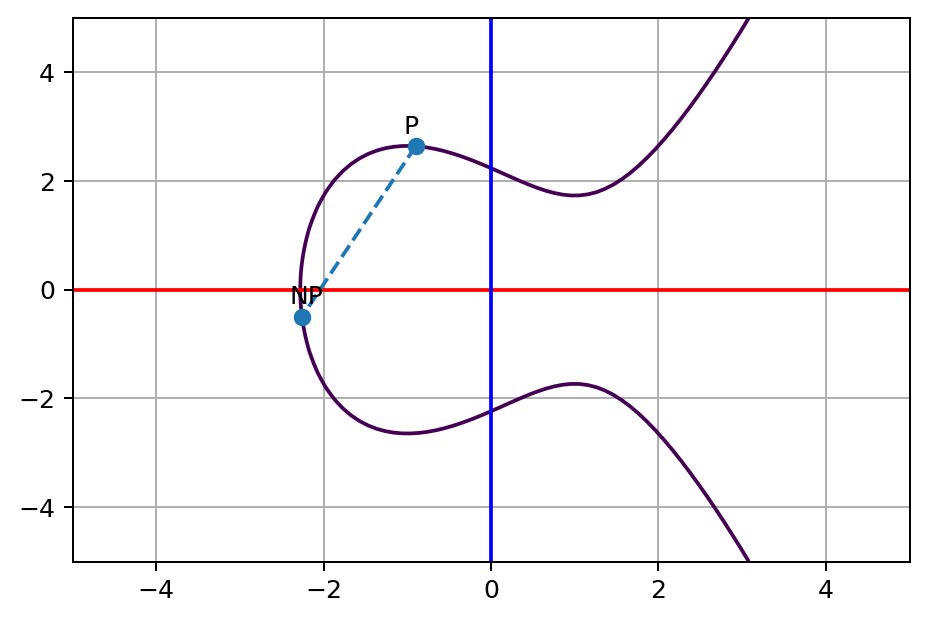given a starting point P and final point NP, can you find what is the N value?

I can tell you the answer $$N$$ of the $$NP$$ point on the diagram above is $$13$$. It’s really easy for me to tell because I pick the number. It would be very hard for you to find out, as there’s no known easy and efficient way to figure out the $$N$$ value.

That’s it, you’ve just learned the basis of Elliptic Curve Cryptography! The curve and original point $$P$$ is a shared value everybody knows and agreed to use, the final point $$NP$$ is your public key, safe to share to anyone. How many steps you jump, the value $$N$$ is your private key. As we said, people who know just $$NP$$ and $$P$$, it will be very hard for them to find out what’s your private key as this is known to be a hard problem to solve.

Not so fast!

I heard you yelled at me.

To get the point NP, doesn’t that mean you need to do it N times. If you can do it in a reasonable amount of time, can’t I just do the same, move forward one by one until I meet the same point NP to find out exactly how many steps it takes?

That’s a great question, I actually got the same one after reading many articles online, but found a few of them explain that clearly. So, next, let’s talk about how to really make your enemies lose track of you in the space.

## Move forward in warp speed

The trick we did to jump around curve for cutting loose enemies, it’s not wise to do it slowly, as it’s easily traceable. Your enemies can simply do the same until they figure out how many times it takes to get there. To really make you untraceable, you need to move forward in warp speed.A clip from Star Trek, spacecraft is warping

The thing is, for the sum operation, or the trick we did on the elliptic curve got an interesting characteristic. The points on the curve and the sum operation follow group law. The idea is, you can apply some kind of operation on the elements in a group, let’s call it “add” here, they will still stay in the group. And the operation comes with specific properties. One of the specific property associativity is like this:

$(A + B) + C$

is the same as

$A + (B + C)$

The mathematic proof behind this concept is actually not easy, you can read it here or here if you are interested. While it’s hard to prove, but it’s easy to see it yourself if you plot the curve and lines. Let’s see an example. As you see we have a point $$(A + B) + C$$ above, according to the associativity property, we should be able to do $$B + C$$ first then $$A + (B + C)$$, and it should arrive at the same final position. Now here’s $$B + C$$.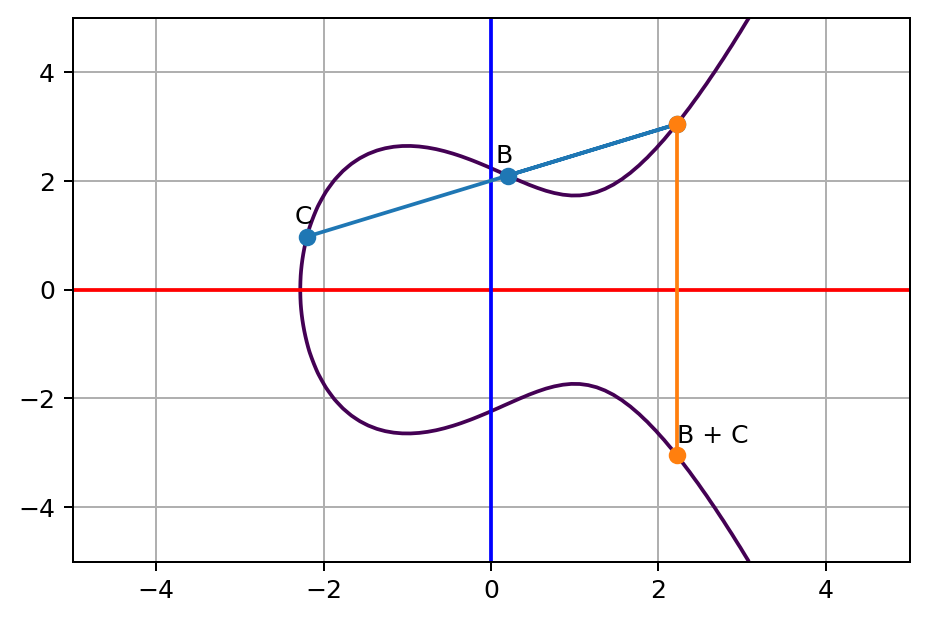B and C line touches a third point on the curve, and its opposite point on the other side of x axis

Next, let’s do $$A + (B + C)$$.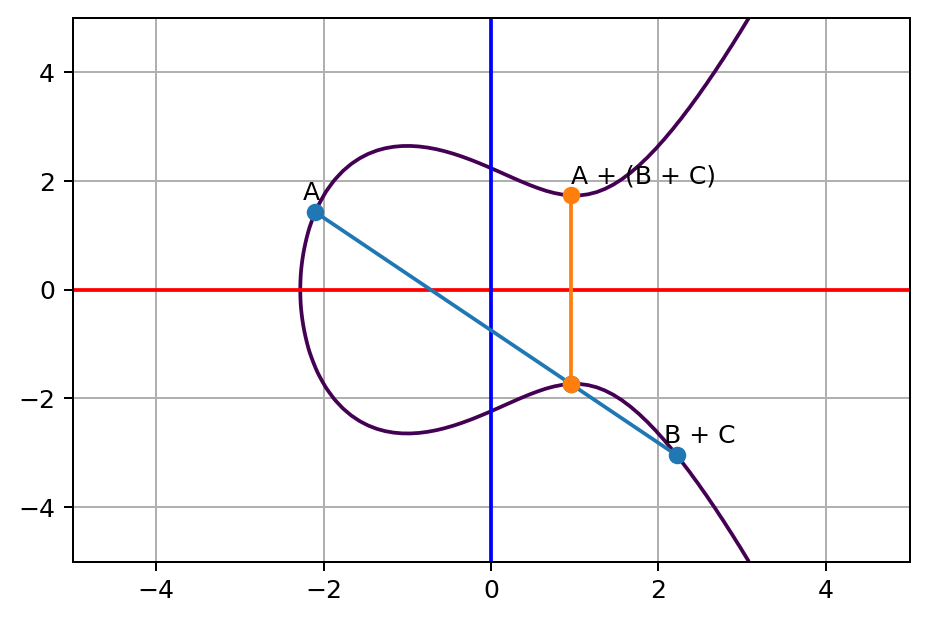A and (B + C) line touches a third point on the curve, and its opposite point on the other side of x axis

Look at the final position, it’s exactly the same as $$(A + B) + C$$. Don’t believe me? Here’s the value printed out from the program I wrote:

ab_c = ab + c
a_bc = a + bc
(ab_c, a_bc)

(Point(0.9531851331698311, 1.733918191357413),
Point(0.9531851331698316, 1.7339181913574133))


As you can see, ab_c and a_bc are mostly identical. The difference is due to floating-point calculation roundoff error. This is actually a big problem, we will talk about this later.

Similarly, for the single-point situation, the group law allows us to sum up to the same position by a different order. Here’s the critical one, remember how we get to $$3P$$ via $$P + 2P$$? Now I want to show you that

$P + 3P = 4P$

is the same as

$2P + 2P = 4P$

At first, let’s see doing the calculation in a silly way, just keep adding $$P$$. Here’s the final step $$P + 3P$$: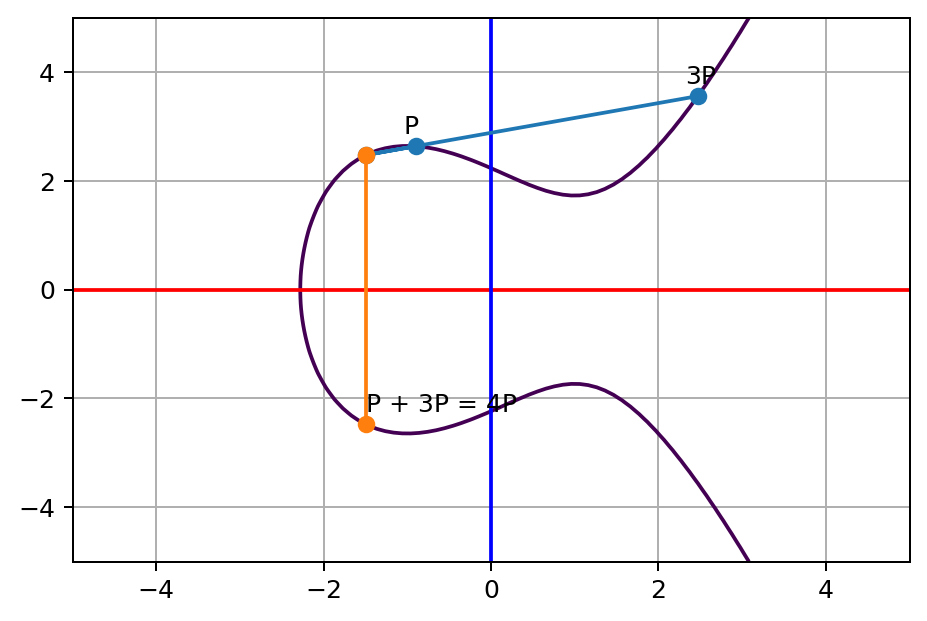P and 3P line touches a third point on the curve, and its opposite point on the other side of x axis

Then, let’s try to add the point $$2P$$ to itself, which is exactly the cutting tangent line trick we did before: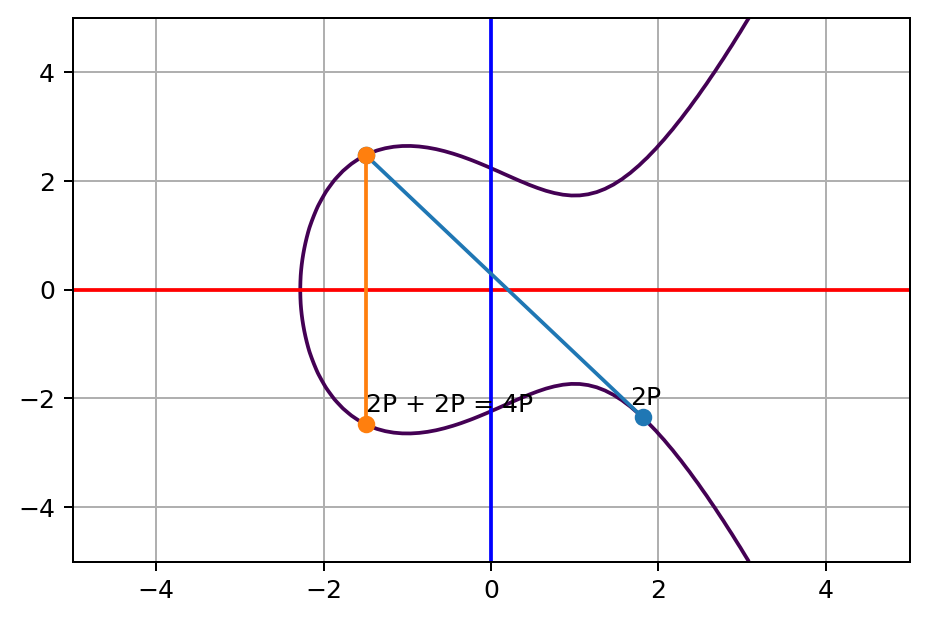2P tangent line touches a third point on the curve, and its opposite point on the other side of x axis

See? Both ways lead to the same result. This is it, since we can double a point easily, and the order of adding it doesn’t matter. We can “cheat” by keep doubling a point and pick the power of the point we need to composite the desired value quickly without adding it up one by one.

Let’s try to add up to a slightly bigger number, say $$227P$$, let’s first break it down to binary so that we can get its power of two composition. Its binary representation is

$11100011$

in other words, summing up all the power of two values would be

$2^{7}P + 2^{6}P + 2^{5}P + 2^{1}P + 2^{0}P$

and i.e

$128P + 64P + 32P + 2P + P$

So the operation we need would be

• Add P and double P
• Add 2P and double 2P
• Double 4P
• Double 8P
• Double 16P
• Add 32P and double 32P
• Add 64P and double 64P
• Add 128P and double 128P

That’s only 8 steps, compares to 227 steps, it’s way faster. This method is called double and add. It allows us to get to the desired multiple times of point jumping on an elliptic curve pretty fast. The number $$227$$ in this example is small, given that we can approach the number we want with $$O(\log{}n)$$, even the number is as big as the number of atoms in the universe, say $$10^{82}$$, which is around $$2^{275}$$, this method can still finish calculating it in 275 double-and-add steps.

Now we know how to jump forward on the elliptic curve in warp speed, with that, we can jump forward bazillion times easily. While it’s easy for us, this is extremely difficult for the attacker to find out exactly how many times we jump, namely, find out what’s the $$N$$ value from $$NP$$ and $$P$$ point given if $$N$$ is big enough. This is called Elliptic Curve Discrete Logarithm Problem, you can search it if you want to learn more about this topic.

## Let’s meet at a secret coordinate

We have been talking about how to jump around a silly elliptic curve in light speed, but what about encryption? Hang on, we are about to introduce it. And before that, let’s introduce key exchange first.

Think about this, Alice and Bob are traveling in the space, they are about to exchange the location of rebellion’s new HQ. Suddenly, they realized there are drones from the empire are tailing them and intercepting the communication between their spacecraft. To exchange information securely, they agree to meet at a secret coordinate where only both of them could know. But how can they exchange this secret coordinate if the enemy is eavesdropping? Now, Elliptic Curve Cryptography is here to rescue. Here’s what Alice and Bob are going to do:

Alice:

Hey Bob, let’s use P as the starting point, here’s my public key NP

Bob:

P as the starting point sounds good to me, and my public key is MP

Here, as how we defined it, $$NP$$ is the point of $$P$$ after $$N$$ times of sum operation. And likewise, $$MP$$ is point of $$P$$ after $$M$$ times of sum operation.

Next, Alice takes Bob’s $$MP$$, start adding this point to itself $$N$$ times:

$\underbrace{MP + MP + ... + MP}_\text{N times} = N \times MP$

Bob will instead take Alice’s public key $$NP$$ and add this point to itself $$M$$ times:

$\underbrace{NP + NP + ... + NP}_\text{M times} = M \times NP$

Well, both $$M$$ and $$N$$ are very big, as we don’t want the enemy to find it out easily. Obviously, they aren’t really adding one-by-one, they are cheating by using the double-and-add trick we just introduced. Eventually, they will meet at a secret coordination $$SK$$ only they could know

$SK = (N \times M)P = (M \times N)P$

Think about this, for Alice, each jump is $$M$$ times worth of point $$P$$, and she does it for $$N$$ times. And for Bob, each jump is $$N$$ times worth of point $$P$$, and he does it for $$M$$ time. Let’s say Alice is jumping 4 lightyears a time, and she jumps 3 times. And Bob is jumping 3 lightyears a time, and he jumps 4 times, $$4 \times 3$$ or $$3 \times 4$$ they are all going to end up at $$12$$: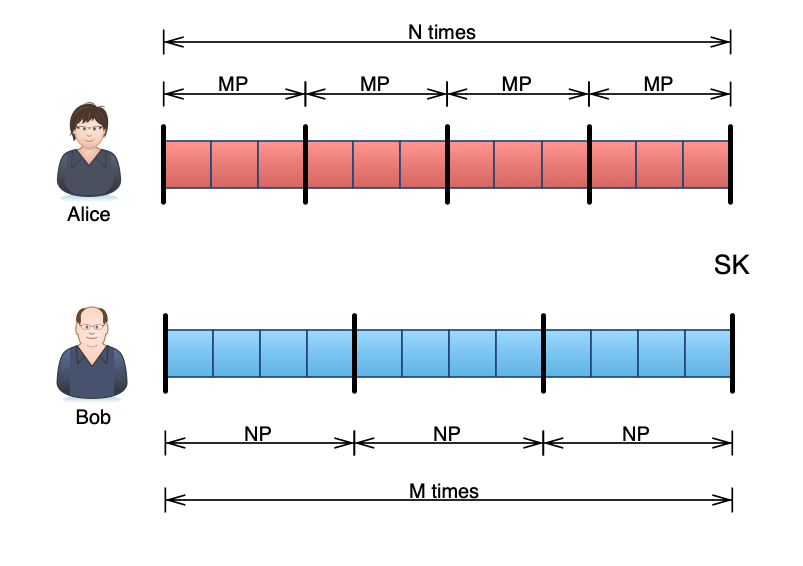Jump 4 points 3 times or jump 3 points 4 times are the same

For eavesdroppers, they need to find out $$N$$ or $$M$$ to be able to get the same coordinate. One step off and the final point will be completely off. But as we mentioned previously, there’s no easy way to do that given the number is big enough, so that we can be sure only Alice and Bob could know this secret coordinate. This key exchange protocol is called Elliptic Curve Diffie–Hellman.

## Encryption

Now let’s talk about encryption. For Alice, to send information securely to Bob, they need to perform Elliptic Curve Diffie–Hellman key exchange first as we just mentioned above:Alice and Bob perform ECDH for key exchange and get shared key SK

Please note here, Alice needs to be able to verify that the public key $$MP$$ really belongs to Bob. Otherwise, an imposter could provide their own public key to Alice, and claim to be Bob. Alice will then exchange a shared secret key with the attacker. The attacker can then perform man-in-middle attack. To address the problem, there’s another concept called Public key infrastructure, as it’s off-topic, you can search it if you’re interested.

As today we want to only focus on Elliptic Curve Cryptography, let’s assume Alice already knew $$MP$$ and have good faith that it’s from Bob, and Bob also knew Alice’s public key. Now, after the key exchange, they end up getting the same shared secret coordinate, we can take the x value as the key. Once we have a shared secret, it’s now easy. We can then use any secure symmetric encryption method to encrypt our secret data with the shared key $$SK$$. Let’s say we use AES256 here, for example. The receiver Bob can decrypt the encrypted message with the same shared secret key $$SK$$.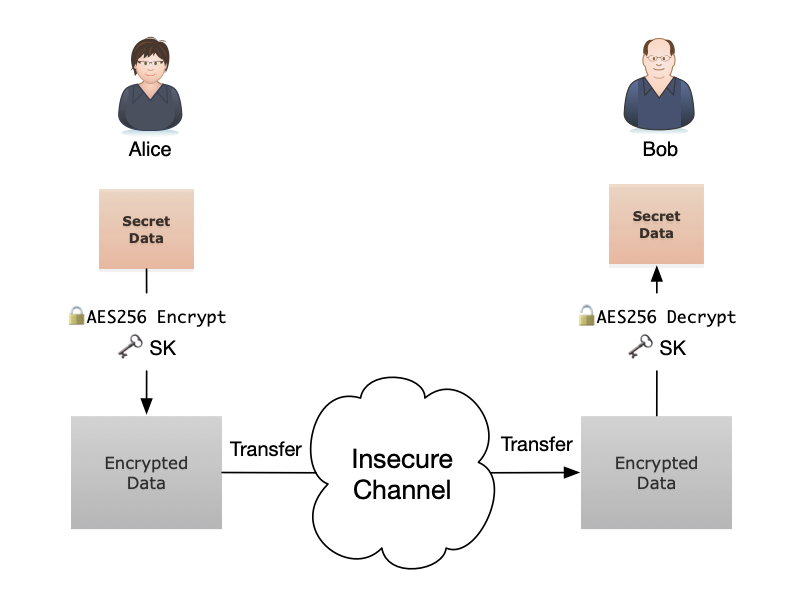Alice encrypts the secret message with shared secret key SK and sends to Bob, Bob then decrypts it with shared secret key SK

Great, now even with drones from empire eavesdropping all the communications, Alice can still share the location of rebellion’s new HQ securely to Bob. Likewise, Bob can use the same shared secret key $$SK$$ to encrypt a secure message and send it back to Alice.

When Alice and Bob know each other, we know we can use Elliptic Curve Cryptography to send a message securely. However, what if there’s a case we want to send a message to someone securely, but they don’t know and probably don’t care who you are? Easy, in our example, we assume Bob already knew Alice’s public key, say if only Alice knows Bob’s public key $$MP$$, she can take her own private key $$N$$, generate the same shared secret key $$SK$$, encrypt the data and send her public key $$NP$$ in plain text along with the encrypted data. Once Bob received the message, he can use the $$NP$$ and his private key $$M$$ to create the same secret key $$SK$$ and decrypt the encrypted data. Just, however, Bob won’t be able to know the message is from whom, as the attached public key $$NP$$ could belong to anyone. Alice can also choose to create a temporary new pair of key for the same operation if Bob doesn’t care who the sender is.

## The problem of floating-point

So far, we have been talking about Elliptic Curve Cryptography with real number calculations. The reason we use real numbers here is that it’s easier to explain and understand. In the real world, this actually isn’t how we do cryptography. Using real number bring many problems, one big problem we have shown previously is that there will be calculation errors. Another problem is, for some extreme cases, the number could be extremely huge, and floating-point may not be able to hold it.

What should we use instead you may ask, the answer is to perform it on finite field, or more accurately, on a finite field of integers modulo p, where p is a prime number. Again, we don’t want to dig the rabbit hole too deep, so if you are interested, you can read Elliptic Curve Cryptography: finite fields and discrete logarithms, or watch this series of video from Trustica and their corresponding articles.

To explain elliptic curve on finite field of integers modulo p in math:

$y^{2} \equiv x^{3} + a x + b\pmod{p}$

And let’s pick $$p = 19$$ and let $$a = -3$$ and $$b = 5$$, then visualize it: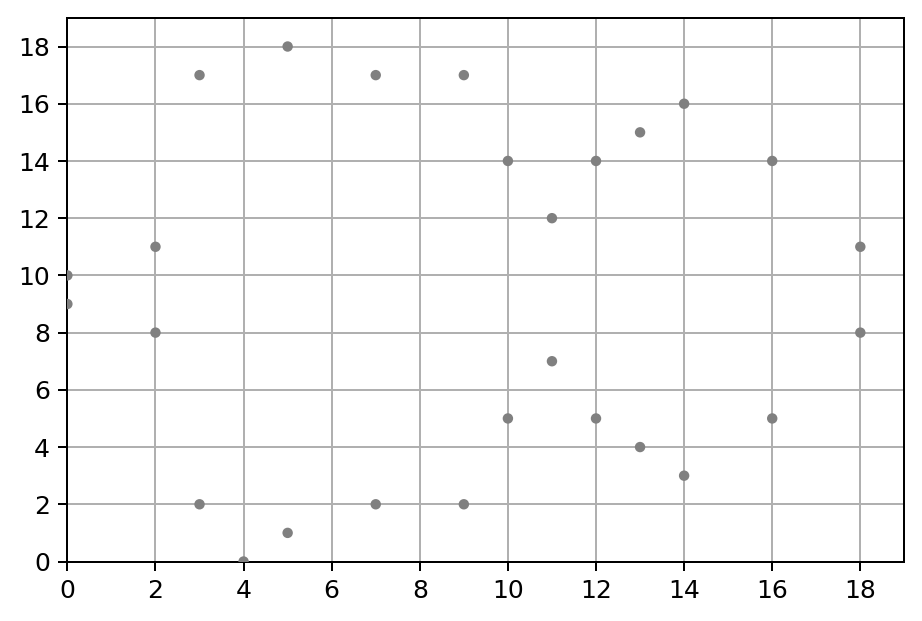Elliptic Curve on finite field of integers modulo p = 19

This doesn’t look like too much of a “curve”, but it’s indeed what an elliptic curve looks like on a finite field. Basically, $$y^{2}\pmod{p}$$ will be equal to $$x^{3} + a x + b\pmod{p}$$ only on specific integer points. Take point $$(11, 7)$$ as the example, for $$y$$:

$y^{2} \equiv 7^{2} \equiv 49 \equiv 11 \pmod{19}$

and for $$x$$:

$x^{3} + a x + b \equiv 11^{3} - 3 \times 11 + 5 \equiv 1303 \equiv 11 \pmod{19}$

As the both sides have the same remainder $$11$$ divided by $$19$$, so that’s indeed a point on the curve.

While it doesn’t look like a curve, but it does follow the same group law like the one on real numbers. Let’s see an example, set point $$A = (3, 2)$$ and $$B = (5, 18)$$, and calculate $$A + B$$ with the same sum operation: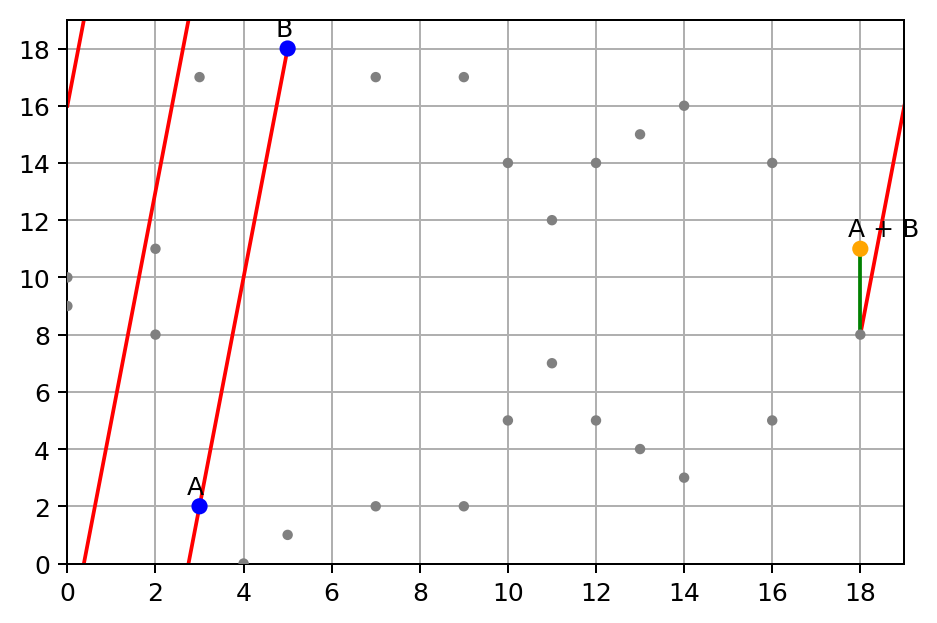Elliptic Curve on the finite field of integers modulo p = 19, sum point A + B

Yep, also, a bit special about this curve on a finite field, when a line hits the boundaries, it can actually warp around to the other end, as the modular operation is circling around. The line will hit the third point somewhere, just like the curve on the real number does.

Given our example, $$(18, 8)$$ is the point we hit. And we flip $$y$$ value $$8$$ to be $$-8$$ and mod by $$19$$ will get you $$(18, 11)$$. So the sum of $$A$$ and $$B$$ is $$(18, 11)$$.

To make it easier to understand, personally I really like the presentation of it in 3D space as a donut shape, like the one from Trustica video series about ECC: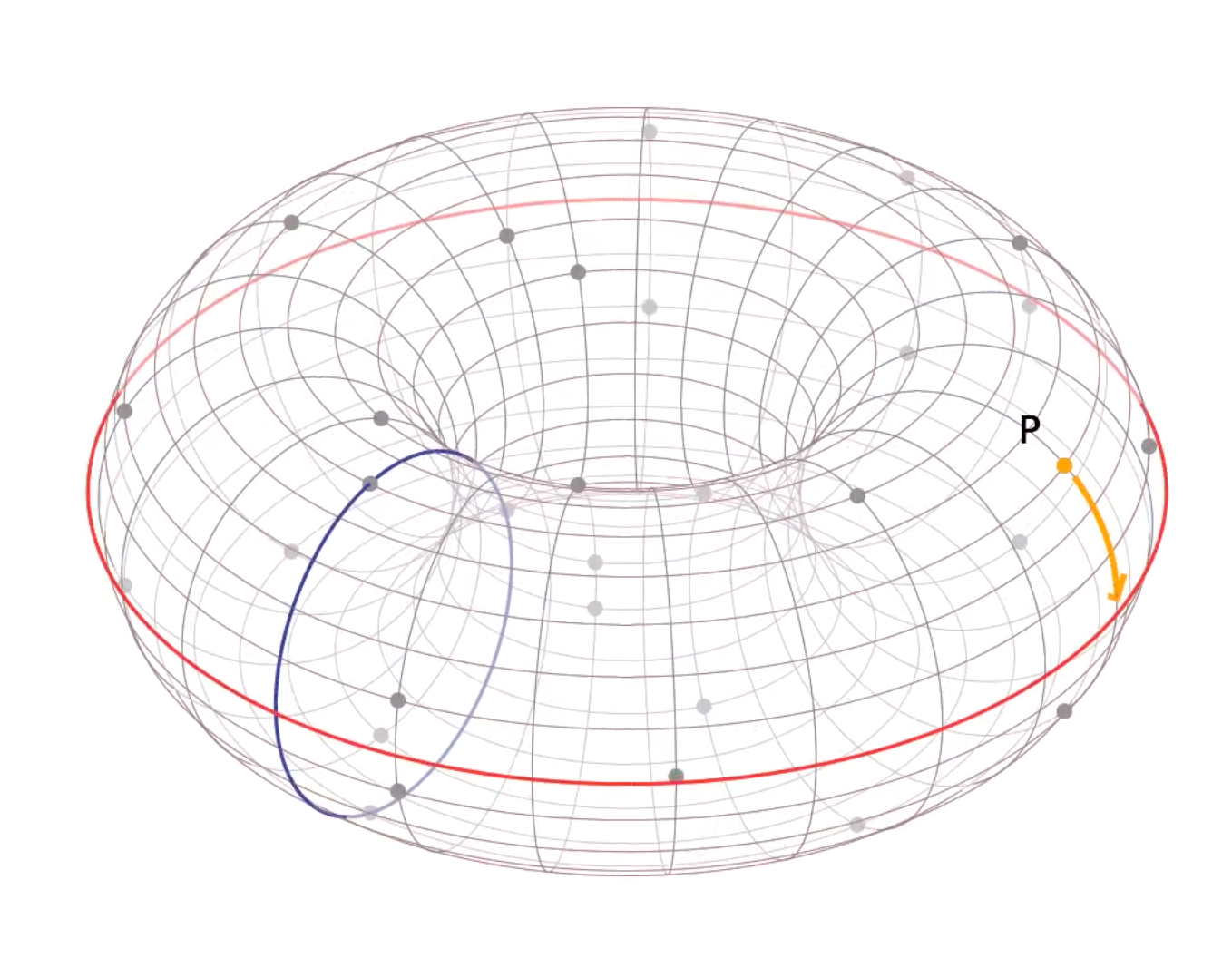Elliptic Curve on finite field in 3D space a donut shape, from this series of video of Trustica

But given that I am writing an article, it’s easier to present it in a simple 2D image.

Now, let’s add a new point $$C = (10, 14)$$ to $$A + B$$: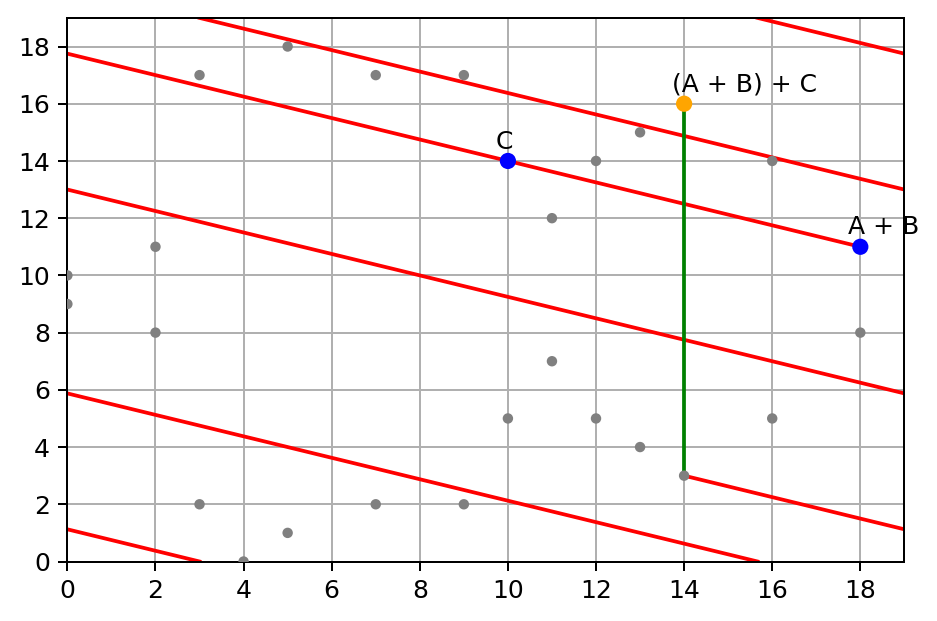Elliptic Curve on finite field of integers modulo p = 19, sum point (A + B) + C

Next, let’s see if associativity of group law still holds with finite field, this time we add $$B + C$$ first: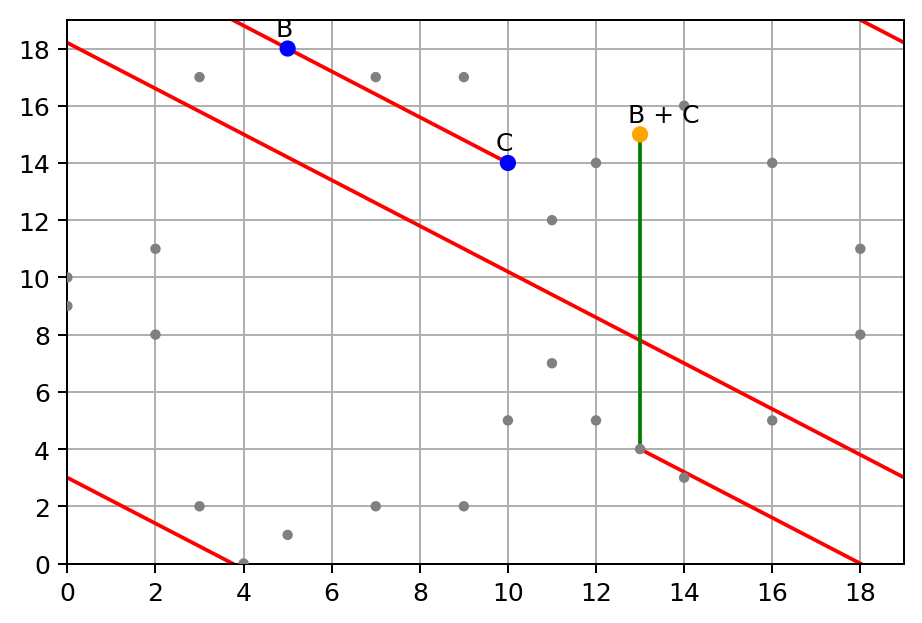Elliptic Curve on finite field of integers modulo p = 19, sum point B + C

Then, let’s add $$A$$ to $$B + C$$: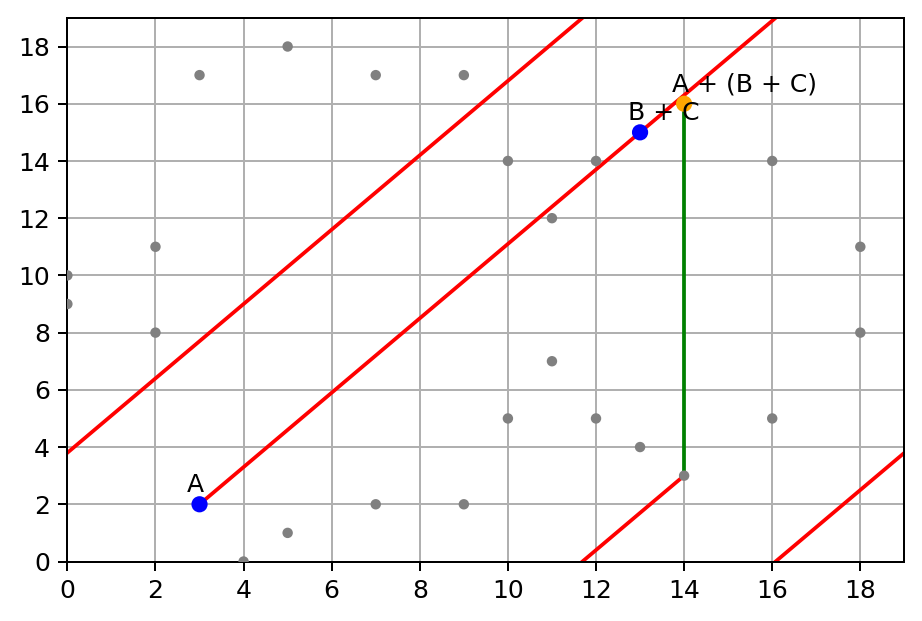Elliptic Curve on finite field of integers modulo p = 19, sum point A + (B + C)

Yep, they both end up at the same point $$(14, 16)$$:

a_b = a + b
b_c = b + c
(a_b + c), (a + b_c)

(Point(p=19, x=14, y=16), Point(p=19, x=14, y=16))


Now you may ask, what about adding a point to itself? Yes, a point adding itself holds the same rule, using the tangent line on the finite field to connect the third. As we’ve already shown how this works with real numbers, we don’t want to repeat ourselves here, or, actually, that’s because I am lazy 😅.

In the end, as the finite field only operates on integers, we won’t lose any precision. It’s more suitable for cryptography purposes.

## Finally

That’s it, now you know how Elliptic Curve Cryptography works. It took me an unexpected level of effort to write this post. If you like my writing, the best way to support me is to follow me on Twitter, dev.to or Medium for new posts, share this article, or buy my book. Feedbacks are welcome. Finally, hopefully, it is as I promised this is intuitive, and you found it helpful. 😊